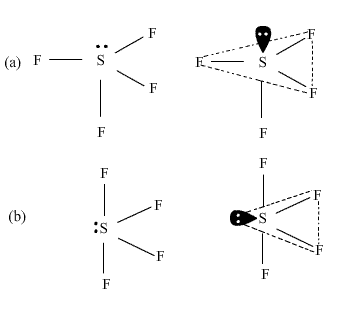# Among the following structures of SF4 which one is more stable and why?

Among the following structures of SF4 which one is more stable and why? What is this shape called?In figure (a), the lone pair of electrons is present at the axial position so there are three lone pair-bond pair repulsions at 900. In the figure(b), the lone pair of electrons is present at the equatorial position and there are two lone pair- bond pair repulsions. Hence figure (b) is more stable as compared to figure (a). The shape shown in figure (b) is distorted tetrahedron, a folded square or a see saw.

1 Like